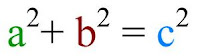### Pythagoras

Thursday, February 26, 2009
Apparently, Mr. Harbeck slipped on ice and bumped his head. Trust me, it happens everywhere in the winters of Winterpeg. That accident has caused him to have amnesia. Now, as a class, he needs us to explain the artifacts he found in his backpack.

-NOTE- Pay attention to the words that are PURPLE because those words are related with the artifacts found with Mr. Harbeck.

1st ArtifactThis shape is called a right angle triangle, R.A.T. for short. The bottom left corner is a right angle, or a 90 degrees angle. The lines connected to it are called legs. These legs are usually labeled "A", and "B". "A" is usually the one that stands upright because it stands for altitude, and "B" is usually on the bottom because it stands for base. However, it doesn't matter, it could work either ways. The longest side of the triangle is called the hypotenuse, and labeled side "C". You could find the hypotenuse across from the right angle. The two angles connected to the hypotenuse are labeled Theta and Beta. These two angles added together equal 90 degrees. That makes those two angles called complimentary angles.2nd Artifact

This artifact is called a square. What makes it a square is the lines of symmetry. The lines of symmetry are the lines that go through each side of a square. This indicates that all sides of the square is equal. Each corner of a square is 90 degrees angle. If you add up all of the corners you will get 360 degrees. Which means that this square is 360 degrees. If you cut this square in half from corner to corner, you will have two R.A.T.'s.

3rd ArtifactThis is the pythagorean theorem. It was created by a guy named Pythagoras. I will talk more about him soon. Anyways, he came up with this equation to find the unknown side of a right angle triangle. Say we know side A, and B of a triangle. What we wanted to find out was C. All we have to do is use the pythagorean theorem. First we square side A and B. Next you add both of them up. And lastly, you find the square root of the sum on the left side, and you find the square root of the C squared on the other side. The number that your left with, should equal to side C.

4th ArtifactThis is a statue of the famous Pythagoras. This is a Greek man that created the pythagorean theorem. He also came up with many other conclusions and theories. Because of this, he is often called the father of math. He figured out that the earth revolves around the sun, and the circumference of the earth. He spent a lot of time in Egypt, and he was the only westerner to be buried in a pyramid. He was also fluent in Egyptian language. Another interesting fact was that Pythagoras was a vegan. A vegan is someone that only eats non-living things.

Here are the two videos Joseph, Adrian and I made.

Thanks for reading and watching, please comment if I missed anything. Thanks and goodbye!